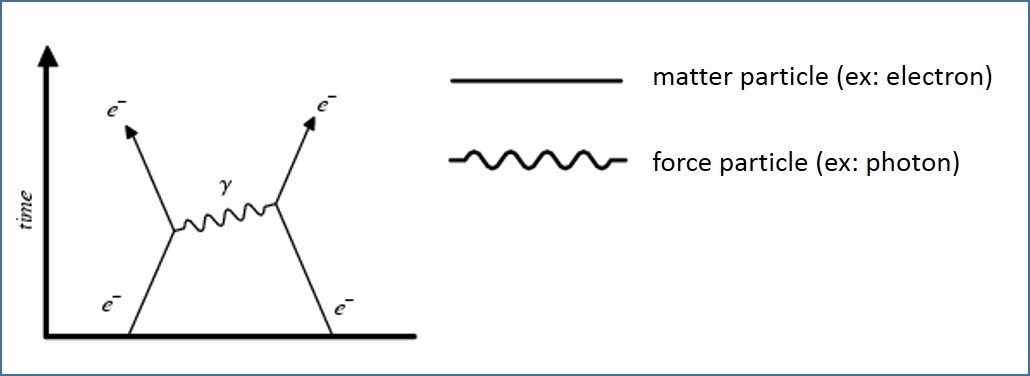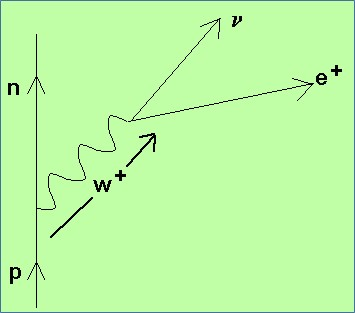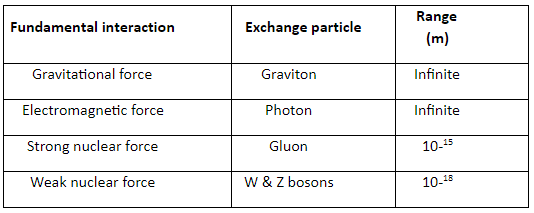# Particle Interactions

## Introduction

The fundamental particle interactions, also known as fundamental forces, are the interactions that do not appear to be reducible to more basic interactions. There are four fundamental interactions known to exist:

• Gravitational force
• Electromagnetic force
• Strong nuclear force
• Weak nuclear force.

The modern quantum mechanical view of the fundamental forces, other than gravity, is that particles of matter do not directly interact with each other, but rather carry a charge and exchange virtual particles, which are the interaction carriers or force mediators. In this article, the four fundamental interactions and exchange particles involved in these interactions are explained. Moreover, a graphical representation of these interactions called the Feynman diagram is discussed.

A. Gravitational Force

Gravitation is by far the weakest of the four interactions at the atomic scale, where electromagnetic interactions dominate. The graviton is the exchange particle for gravity force. Although graviton has not been directly observed, a number of its properties can be implied from the nature of the force. Since gravity is an inverse square force of apparently infinite range, it can be implied that the rest mass of the graviton is zero.

B. Electromagnetic Force

Electromagnetic force is the interaction that occurs between electrically charged particles. The virtual photon (or boson) is the exchange particle responsible for the electromagnetic force. This phenomenon includes the electrostatic force acting between charged particles at rest, and the combined effect of electric and magnetic forces acting between charged particles moving relative to each other.

Electromagnetic force is infinite-ranged, like gravity, but vastly stronger. Electromagnetic force fundamentally determines all macroscopic, and many atomic-level, properties of the chemical elements, including all chemical bonding.

C. Strong Nuclear Force

Strong nuclear force exists in the nucleus in order to keep the elements of the nucleus against their repulsive force. Gluons are the exchange particles in strong nuclear interactions.

D. Weak Nuclear Force

The weak nuclear force acts within the nucleus, quarks and leptons and excerpt forces on each other by exchanging bosons. The weak interaction or weak nuclear force is responsible for some nuclear phenomena such as beta decay. According to the theory of the weak nuclear interaction, the carriers of the weak force are the gauge bosons called the W and Z bosons.

E. Feynman Diagrams

Feynman diagrams are visual representations of particle interactions, which also show the exchange of particles involved. Feynman diagrams are mathematical expressions describing the behaviour of subatomic particles. A simple Feynman diagram that represents a repulsive force between two electrons is illustrated in Figure 1.Figure 1: Feynman Diagram

Each line is called a particle. The vertex is called an interaction. The lines with arrows are matter particles (fermions). The wiggly line is a force particle (boson) which, in this case, mediates electromagnetic interactions: it is the photon. The y-axis in a Feynman diagram represents time.

Examples of a Feynman Diagram:Figure 2: βDecayFigure 3: β+ DecayFigure 4: Electron CaptureFigure 5: Electron – Proton Collision

G. Summary

• The four fundamental interactions known to exist are gravitational force, electromagnetic force, strong nuclear force and weak nuclear force.• A graviton is the exchange particle for gravity force.
• A virtual photon (or boson) is the exchange particle responsible for electromagnetic force.
• Gluons are the exchange particles in strong nuclear interactions.
• Carriers or exchange particles of the weak nuclear force are the gauge bosons called the W and Z bosons.
• Feynman diagrams are visual representations of particle interactions, which also show the exchange of particles involved.